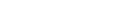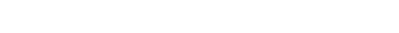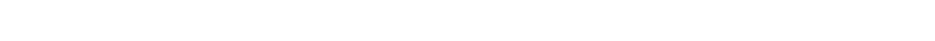# Markowitz Portfolio Theory: FA4

## Markowitz Portfolio Theory

In this part of our financial analytics series, we will be studying Markowitz portfolio theory.  If you have not followed this series before, please check part 1, part 2 and part 3 to learn more about financial analytics.

Portfolio theory published by Harry Markowitz in 1952 helps us in the formulation of great portfolio theory which forms base of financial analytics.
Following are the highlights of this theory:

• Rather than putting everything on a single place as one investment, he emphasized on creating a diverse portfolio from different industries and then optimizing the risk involved in it.
• These industries shouldn’t be having correlations between them or the correlations between them should be minimum in order to prevent additional risks from coming up.
• This optimized portfolio leads to higher returns also.

### Financial Analytics Case-study

In order to understand this, let’s get into a case-study first.

Let’s assume there are two shares:

• Share X – Expected return = 8%; Standard deviation = 4%
• Share Y – Expected return = 10%; Standard deviation = 3%

The correlation between share X and share Y = 0.2 or 20%. We know that 0.2(20%) is very far away from 1(100%). The positive correlation denotes that as share X increases, share Y also increases and as share X decreases, share Y decreases but this relation is very small.### Expected return

Let’s now work on finding the Expected return and Standard deviation for each of the portfolios.
Formula for expected return is as follows:Thus, we get the following table as the output after applying this formula:Evaluating this, we get the following values of expected returns:### Standard deviation

The formula of standard deviation of a portfolio is as follows:We have

• Share X – Expected return = 8%; Standard deviation = 4%
• Share Y – Expected return = 10%; Standard deviation = 3%
The correlation between share X and share Y = 0.2 or 20%

Thus, we get the following table:Evaluating this, we get the following table:After taking square root of the last column, we get the following table:In percentage, the output becomes:In order to get maximum expected return and minimum standard deviation (risk), we will choose portfolio 4 which is giving us expected return of 9.5% and standard deviation of 2.6%.

One thing to be noticed over here is since the share Y has more expected return and less standard deviation, as we increased weight towards share Y, we got increased expected return and decreased standard deviation. But, generally, in real-world, shares of high expected return involve high standard deviation and that of low expected return involve low standard deviation. So, in real-world, we would be experiencing both of them moving high together.

So guys, with this I conclude this tutorial. In the next part of this series, we wil be implementing Markowitz theory using Python. If you want to learn such things via videos, checkout our YouTube channel – ML for Analytics. Stay tuned.